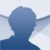# Derive

5

## Derive works with symbolic annotation. Download Derive and discover one of the best symbolic mathematic calculation applications, like Mathematica or Maple

Unlike numerical computation applications like MATLAB or Scilab that use approximate real values to perform the mathematical operations, symbolic mathematics programs allow you to perform the calculations using the number's exact value because they make use of what's called symbolic computation.

### An intuitive application to learn mathematics

Some of the best known symbolic mathematics applications are Mathematica, Maple and Derive. This last one is one of the most used in university environments due to how easy it is to use. It's a powerful mathematics program developed to operate with symbolic notation, even though it also allows numerical computation.

Derive is capable of solving derivatives, integrals, limits, series, vector and matrix operations, polynomials, algebraic fractions,... Furthermore, it has several options for the graphical representation of bidimensional and tridimensional functions. The result of the operations performed with Derive will be an exact value, expressed with a finite number of decimals, with the precision chosen by the user.